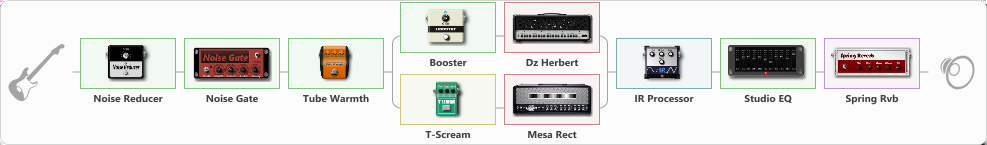# Mesa Diezel blend

Discussion in 'ToneLib-GFX presets' started by Tony the Metalhead, May 4, 2022.

1. ### Tony the MetalheadActive Member

Mesa Diezel blend

Preset name: MESA/DIEZEL

Effects chain:Effect: "Noise Reducer" (Dynamics / Filter), active - "yes"
{
"Sens" = 20
"Mode" = Soft
}

Effect: "Noise Gate" (Dynamics / Filter), active - "yes"
{
"Mode" = Auto
"Depth" = 50
"Threshold" = 50
"Attack" = 0
"Hold" = 5
"Decay" = 250
}

Effect: "Tube Warmth" (Dynamics / Filter), active - "yes"
{
"Input" = 80
"Warmth" = 25
"Level" = 100
}

Effect: "Splitter" (Dynamics / Filter)
{
"A-Bypass" = Off
"A-Pan" = 0
"A-Level" = 55
"B-Bypass" = Off
"B-Pan" = 0
"B-Level" = 60
"Width" = 0

'A' branch:
{

Effect: "Booster" (Dynamics / Filter), active - "yes"
{
"Gain" = 100
}

Effect: "Dz Herbert" (Amp simulators), active - "yes"
{
"Gain" = 50
"Bass" = 20
"Middle" = 70
"Treble" = 50
"Presence" = 55
"Master" = 80
"Level (dB)" = 0
}
}
'B' branch:
{

Effect: "T-Scream" (Overdrive / Distortion), active - "yes"
{
"Drive" = 10
"Tone" = 80
"Level" = 80
}

Effect: "Mesa Rect" (Amp simulators), active - "yes"
{
"Gain" = 40
"Bass" = 40
"Middle" = 0
"Treble" = 60
"Presence" = 40
"Master" = 85
"Level (dB)" = 0
}
}
}

Effect: "IR Processor" (Cabinets), active - "yes"
{
"IR" = hardwire_LR_Stereo
"Low Cut (Hz)" = 80
"Hi Cut (kHz)" = 16.0
"Mix" = 100
"Level (dB)" = 0
}

Effect: "Studio EQ" (Dynamics / Filter), active - "yes"
{
"31 Hz" = -6
"62 Hz" = -5
"125 Hz" = -4
"250 Hz" = -2
"500 Hz" = -4
"1 kHz" = -2
"2 kHz" = 2
"4 kHz" = 4
"8 kHz" = 6
"16 kHz" = 10
"Level (dB)" = 0
}

Effect: "Spring Rvb" (Reverberation), active - "yes"
{
"Time" = 3.0
"PreDelay" = 0
"LoDamp" = 10
"HiDamp" = 60
"Mix" = 20
}

Note: You will need to download and install the ToneLib-GFX software to use the preset.

#### Attached Files:

• ###### Mesa_Diezel_blend.tlgfx
File size:
2.1 KB
Views:
2,131
N8tdy likes this.